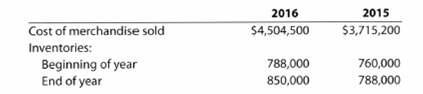Chapter 7, Problem 7.8APE### Accounting (Text Only)

26th Edition
Carl Warren + 2 others
ISBN: 9781285743615

#### Solutions

Chapter
Section### Accounting (Text Only)

26th Edition
Carl Warren + 2 others
ISBN: 9781285743615
Textbook Problem
1 views

# Inventory turnover and number of days’ sales in inventorya. Determine the inventory turnover for 2016 and 2015. b. Determine the number of days ‘sales in inventory for 2016 and 2015. Use 365 days and round to one decimal place. c. Does the change in inventory turnover and the number of days’ sales in inventory from 2015 to 2016 indicate a favorable or an unfavorable trend?

(a)

To determine

Inventory turnover ratio: Inventory turnover ratio is used to determine the number of times inventory used or sold during the particular accounting period. The formula to calculate the inventory turnover ratio is as follows:

Inventory turnover=Cost of goods soldAverage inventory

Days’ sales in inventory: Days’ sales in inventory are used to determine number of days a particular company takes to make sales of the inventory available with them. The formula to calculate the days’ sales in inventory ratio is as follows:

Days' sales in inventory=Days in accounting periodInventory turnover

inventory turnover for 2016 and 2015.

Explanation

The inventory turnover ratio is calculated by dividing cost of goods sold by average inventory during the period. The average inventory is calculating by dividing beginning inventory and ending inventory by 2...

(b)

To determine

Days’ sales in inventory ratio for 2016 and 2015.

(c)

To determine

To explain: if change in turnover and days’ sales in inventory from 2015 to 2016 indicate favorable or unfavorable trend.

### Still sussing out bartleby?

Check out a sample textbook solution.

See a sample solution

#### The Solution to Your Study Problems

Bartleby provides explanations to thousands of textbook problems written by our experts, many with advanced degrees!

Get Started